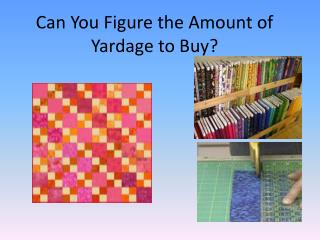DownloadDownload PresentationCan You F igure the Amount of Yardage to Buy?

Can You F igure the Amount of Yardage to Buy?

Télécharger la présentationCan You F igure the Amount of Yardage to Buy?

- - - - - - - - - - - - - - - - - - - - - - - - - - - E N D - - - - - - - - - - - - - - - - - - - - - - - - - - -
Presentation Transcript

1. Can You Figure the Amount of Yardage to Buy?

2. What size is your bed?

3. Different sizes need different fabric requirements: • Twin size bed = 63 X 87” • Double (full) size = 78” X 87” • Queen bed = 84” X 92” • King bed = 100” X 92” • If your quilt pattern fits a twin size bed but you have a queen bed, what can we do to create it big enough for your bed?

4. How Well Do You Know Your Math? • What do we call the different sides of a rectangle? • Length • Width • What is the general shape of a quilt? • Rectangle

5. How many inches in a yard? • 36” • How do you convert 6 ft into inches? • 6(12”) = 72” • What does the perimeter give you? • All the lengths of the sides added together • Does everyone remember the perimeter formula? • Perimeter = 2(w) + 2(l)

6. This quilt is 70 X 86”. • 2(w) + 2(l) = p • 2(70) + 2(86) = 312 inches • If a twin size quilt is 63” x 87”, what is it’s perimeter? • 2(63) + 2(87) = 300 inches • Could this quilt fit a twin size bed? • Could it fit a double or full size bed that is 78” X 87”? 86” 70” • What is the perimeter of this quilt?

7. A queen size bed is 84” x 92” and our quilt pattern is 70” X 86”. • If you add a 6.5 inch border to your quilt, could it fit a queen size bed? • That would increase the size 6 inches because the seams are a quarter inch. 1/4 inch seam on the inside and outside edges equals 2/4 or 1/2. • With Border, the quilt will be 82” x 98”. New border added Queen size Original quilt

8. NOW, how much fabric do we need to purchase for the border? 70” 86” 6.5 “ border • Find the perimeter plus the extra border length • P = 2(w) + 2 (l) + 4(b) p = 2(70) + 2 (86) + 4(6.5)= 338 (total length)

9. A bolt of fabric is often called 45” wide fabric but it can vary in width from 42” to 45”. (the most common width is 44”) • Now we need to divide the total length by the 43” width of the fabric bolt. • Round up to the nearest whole number. • Total border length/ bolt width • 338”/ 43” = 7.86 strips • So we will round up to 8 strips of 6.5” fabric needed for border.

10. Now we need to figure out how many yards of fabric to purchase. • Multiply the number of strips by the border width. (6.5”) • How many yards should you purchase to cover the 52 inch length? • 8 strips x 6.5” border width = length • 52” / 36” = 52” 1 yard + 16” • round up to 1 ½ yards

11. The same math principal applies to many things. • What is the perimeter of a football field 360 ‘ x 160 ‘. • You are going to put a 4” border around a bulletin board that is  4’ x 6’. • What is the total length of border needed? • 2(l) + 2(w) + 4(b) = • 2(48) + 2(72) +4(4) = 256” • 2(l) + 2(w) = feet 1040

12. Queen King • So….. if you have a King size bed (100”x 92”), how would you now adjust a queen size quilt (84”x92”). to fit a king size bed? 1. What is the border width going to be? 2. What is the total length of border needed? • 1. (100 – 84) / 2 = 8 (8 inch border) • 2. The total length of the border will equal 2(l) = 2(92”)=184”

13. 3. How many linear inches of the 44” wide bolt will we need? 4. How many yards is 40”? 3. The total length of the border will equal 2(l) = 2(92”) =184” 4. 40”/ 36” = 1 yd and 4”. Round up to 1 1/8 yards of fabric needed to make a king size quilt.

14. Home work assignment:- Do #1 – 4 here and then….-Go home and measure one quilt from one of your beds. -Find the amount of fabric needed to purchase to add a border to your quilt.-You determine the size of border you want.